## Sunday, March 11, 2007

### Polar Flips

Sometimes it is necessary to see the deep impact a thought could have as we try to understand the implications of a "sphere dropped in a viscously liquid" that we might find another correlation in how we see the photon affected in the gravitational field. Can we grasped the feeling illicitated to say we have some what of a deep impact in remembering to think the strange world of fluids could entertain us?

Stokes' law

In 1851, George Gabriel Stokes derived an expression for the frictional force exerted on spherical objects with very small Reynolds numbers (e.g., very small particles) in a continuous viscous fluid by solving the small fluid-mass limit of the generally unsolvable Navier-Stokes equations: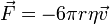where: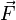is the frictional force,
r is the Stokes radius of the particle,
η is the fluid viscosity, and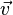is the particle's speed.

If the particles are falling in the viscous fluid by their own weight, then a terminal velocity, also known as the settling velocity, is reached when this frictional force combined with the buoyant force exactly balance the gravitational force. The resulting settling velocity is given by: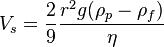where:

Vs is the particles' settling velocity (cm/sec) (vertically downwards if ρp > ρf, upwards if ρp < ρf),
r is the Stokes radius of the particle (cm),
g is the standard gravity (cm/sec2),
ρp is the density of the particles (g/cm3),
ρf is the density of the fluid (g/cm3), and
η is the fluid viscosity (dyne sec/cm2).

Why are Planets Round?

Q9:
Would these fluids act differently on the Moon and at (on) different gravities

I was loosing the train of thought within this post, and then the thought occurred me.

Why are planets round. This is a "general question" which leads to how we see the formation of the planets?

Start there. We also know what a "sphere of water" looks like in space?

While the sphere was being thought of in regards to Stokes's Theorem, I was also thinking of the earth in relation to how we see gravity in regards to the earth already formed. The vicissitude, in which the earth exists within the cosmos. The moon.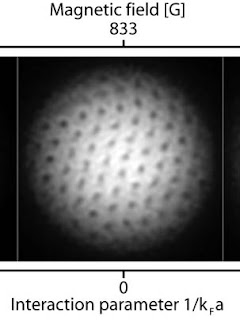The rotating superfluid gas of fermions is pierced with the vortices, which are like mini-tornadoes. Image / Andre Schirotzek, MIT

Bose-Einstein condensation of pairs of fermions that were bound together loosely as molecules was observed in November 2003 by independent teams at the University of Colorado at Boulder, the University of Innsbruck in Austria and at MIT. However, observing Bose-Einstein condensation is not the same as observing superfluidity. Further studies were done by these groups and at the Ecole Normale Superieure in Paris, Duke University and Rice University, but evidence for superfluidity was ambiguous or indirect.

The superfluid Fermi gas created at MIT can also serve as an easily controllable model system to study properties of much denser forms of fermionic matter such as solid superconductors, neutron stars or the quark-gluon plasma that existed in the early universe.There is a special class of fluids that are called superfluids. Superfluids have the property that they can flow through narrow channels without viscosity. However, more fundamental than the absence of dissipation is the behavior of superfluids under rotation. In contrast to the example of a glass of water above, the rotation in superfluids is always inhomogeneous (figure). The fluid circulates around quantized vortex lines. The vortex lines are shown as yellow in the figure, and the circulating flow around them is indicated by arrows. There is no vorticity outside of the lines because the velocity near each line is larger than further away. (In mathematical terms curl v = 0, where v(r) is the velocity field.)

See here for more on Attributes of Superfluids

It is very possible that the information is inundating my thinking here yet without considering the context of the super fluid what requirements would fit the idea that earth is relevant when it comes to the molten core? Or how you see the magnetic field shifting in relation to the poles?

Thus, water is "thin", having a lower viscosity, while vegetable oil is "thick" having a higher viscosity. All real fluids (except superfluids) have some resistance to shear stress, but a fluid which has no resistance to shear stress is known as an ideal fluid or inviscid fluid (Symon 1971).

I am quickly posting this and will have more to add. I wanted to speak directly to the idea of the super fluid. How the "irrotational value of the perfect fluid as a whole," could have it's leakages to the surface of the earth, as small vortices created.This computer simulation shows the Earth's interior as its magnetic field reverses, perhaps because of changes in the flow of molten iron in the core. Deep inside the Earth, the magnetic field arises as the fluid core oozes with hot currents of molten iron and this mechanical energy gets converted into electromagnetism. It is known as the geodynamo. In a car's generator, the same principle turns mechanical energy into electricity.

No one knows precisely why the field periodically reverses, but scientists say the responsibility probably lies with changes in the turbulent flows of molten iron, which they envision as similar to the churning gases that make up the clouds of Jupiter.

In theory, a reversal could have major effects because over the ages many aspects of nature and society have come to rely on the field's steadiness.Quasar posted a comment there that immediately made me think what the topic could mean in relation to the post he was commenting on. I thought of the earth's core as the subject was related, and thought how nice it would be to have such a "cylindrical channel that goes from pole to pole?"

Well the viscosity of the fluid as it traverses this cylinder would give some nature to the charge as it passes through? I do not think it could be that simple, if we thought the iron molten as the viscosity had a direct relation to what we know of our magnetic field? We know the earth core is not so cylindrical, that we could the attribute of the superfluid in this case while looking at the iron molten lava

So is it the iron in particular that gives us our strength based on it's fluid's nature?These changes in Earth’s magnetic fields from 1980 to 2000 may be harbingers of a shift in the magnetic poles